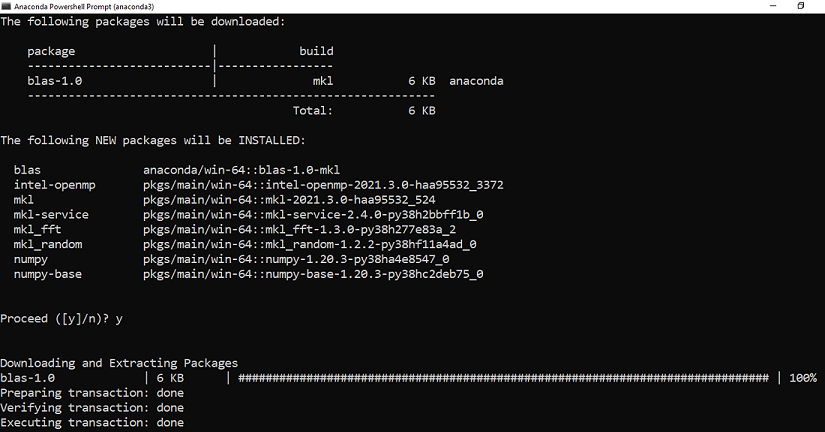GFG App
Open AppBrowser
Continue

# How to Install Numpy on Windows?

Python NumPy is a general-purpose array processing package that provides tools for handling n-dimensional arrays. It provides various computing tools such as comprehensive mathematical functions, linear algebra routines. NumPy provides both the flexibility of Python and the speed of well-optimized compiled C code. Its easy-to-use syntax makes it highly accessible and productive for programmers from any background.

## Pre-requisites:

The only thing that you need for installing Numpy on Windows are:

## Installing Numpy on Windows:

### For Conda Users:

If you want the installation to be done through conda, you can use the below command:

`conda install -c anaconda numpy`

You will get a similar message once the installation is completeMake sure you follow the best practices for installation using conda as:

• Use an environment for installation rather than in the base environment using the below command:
```conda create -n my-env
conda activate my-env```

Note: If your preferred method of installation is conda-forge, use the below command:

`conda config --env --add channels conda-forge`

### For PIP Users:

Users who prefer to use pip can use the below command to install NumPy:

`pip install numpy`

You will get a similar message once the installation is complete:Now that we have installed Numpy successfully in our system, let’s take a look at few simple examples.

Example 1: Basic Numpy Array characters

## Python3

 `# Python program to demonstrate ` `# basic array characteristics ` `import` `numpy as np ` ` `  `# Creating array object ` `arr ``=` `np.array( [[ ``1``, ``2``, ``3``], ` `                ``[ ``4``, ``2``, ``5``]] ) ` ` `  `# Printing type of arr object ` `print``(``"Array is of type: "``, ``type``(arr)) ` ` `  `# Printing array dimensions (axes) ` `print``(``"No. of dimensions: "``, arr.ndim) ` ` `  `# Printing shape of array ` `print``(``"Shape of array: "``, arr.shape) ` ` `  `# Printing size (total number of elements) of array ` `print``(``"Size of array: "``, arr.size) ` ` `  `# Printing type of elements in array ` `print``(``"Array stores elements of type: "``, arr.dtype) `

Output:

```Array is of type:
No. of dimensions:  2
Shape of array:  (2, 3)
Size of array:  6
Array stores elements of type:  int64```

Example 2: Basic Numpy operations

## Python3

 `# Python program to demonstrate ` `# basic operations on single array ` `import` `numpy as np ` ` `  `a ``=` `np.array([``1``, ``2``, ``5``, ``3``]) ` ` `  `# add 1 to every element ` `print` `(``"Adding 1 to every element:"``, a``+``1``) ` ` `  `# subtract 3 from each element ` `print` `(``"Subtracting 3 from each element:"``, a``-``3``) ` ` `  `# multiply each element by 10 ` `print` `(``"Multiplying each element by 10:"``, a``*``10``) ` ` `  `# square each element ` `print` `(``"Squaring each element:"``, a``*``*``2``) ` ` `  `# modify existing array ` `a ``*``=` `2` `print` `(``"Doubled each element of original array:"``, a) ` ` `  `# transpose of array ` `a ``=` `np.array([[``1``, ``2``, ``3``], [``3``, ``4``, ``5``], [``9``, ``6``, ``0``]]) ` ` `  `print` `(``"\nOriginal array:\n"``, a) ` `print` `(``"Transpose of array:\n"``, a.T) `

Output:

```Adding 1 to every element: [2 3 6 4]
Subtracting 3 from each element: [-2 -1  2  0]
Multiplying each element by 10: [10 20 50 30]
Squaring each element: [ 1  4 25  9]
Doubled each element of original array: [ 2  4 10  6]

Original array:
[[1 2 3]
[3 4 5]
[9 6 0]]
Transpose of array:
[[1 3 9]
[2 4 6]
[3 5 0]]```

My Personal Notes arrow_drop_up